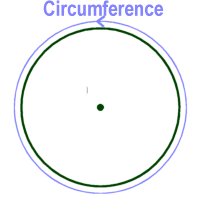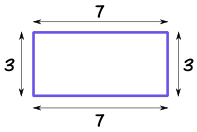# Mathematics Scheme of Work, SS1, 1st Term

### Scheme of Work (Mathematics) SS1, 1st Term:

#### WEEK 1

Topic: Number Bases

• Decimal base (Base 10) and other bases e.g. base 2(binary) base 7 (days of the week) etc.
• Conversion from Base 10 to other bases, conversion from other bases to base 10..

#### WEEK 2

Topic: Number Bases

• Problem solving, addition, subtraction, multiplication and division of number in the various bases.
• Conversion of decimal fraction in one base to base 10.
• Apply number base system to computer programming. .

#### WEEK 3

Topic: Modular Arithmetic

• Revision of addition, division, multiplication and subtraction of integers.
• Concept of modular arithmetic
• Addition, subtraction and multiplication operations in modular arithmetic.
• Application to real life situations.

#### WEEK 4

Topic: Indices

• Laws of indices and their applications e.g. a.  ax x ay = ax+y b.  ax/ay = ax-y c.  (ax)y = axy
• Application of indices, simple indicial/exponential equations.

#### WEEK 5

Topic: Standard Form (AX10n)

•  Writing numbers in index form
• Adding two numbers and writing the results in standard form.
• Subtracting one number from the other in standard form.
• Multiplying numbers in standard form
• Dividing numbers in standard form including square root of such numbers.

#### WEEK 6

Topic: Logarithms

• Deducing logarithm from indices and standard form i.e. if y=10x, then x=logy10
• Definition of logarithm e.g.  log101000=3
• Graph of y=10x using x=0.1, 0.2,………..

#### WEEK 7

Topic: Logarithms

• Calculations involving multiplication and division

#### WEEK 8

Topic: Logarithms

• Calculations involving power and roots using the logarithm tables.
• Solving practical problems using logarithm tables relating to capital market.
•   Explain the concept of capital market operation
• Use logarithm tables in multiplying the large numbers involved in capital market operation.

#### WEEK 9

Topic: Definition of Sets

• Set notation – listing or roster method, rule method, set builder notation
• Types of sets: e.g. universal set, empty set, finite set and infinite set, subset, disjoint set, power set etc.

#### WEEK 10

Topic: Set Operations

• Union of sets and intersection of sets complement of sets.
• Venn diagram
• Venn diagram and application up to 3 set, problems

#### WEEK 11

Topic: Simple Equations

•  Change of subject of formulae
• Formula involving brackets, roots and powers.
• Subject of formula and substitution.

#### WEEK 12

Topic: Simple Equation and Variations

• Revision of simultaneous linear equation in two (2) unknown
• Types and application of variations.

• Revision

#### WEEK 14

• Examination

Mathematics scheme of work, SS1, 2nd Term

Mathematics scheme of work, SS1, 3rd Term

Read topics on Mathematics here

THANKS FOR READING - Please Help Share!

#### NOTABLE POINTS IN Mathematics Circumference in mathematics means the distance around the edge of a circle (or a another curverd surface). Perimeter means the distance around a square or rectangle or polygon. Circumference is to circle or any other curved surfaceImage Credit: Ducksters Perimeter is to square or rectangle or polygonImage Credit: Maths is Fun

CONTRIBUTE TO THIS TOPIC | ASK A QUESTION